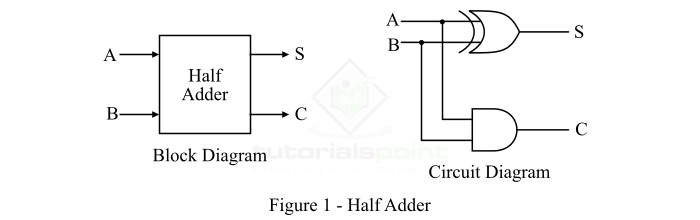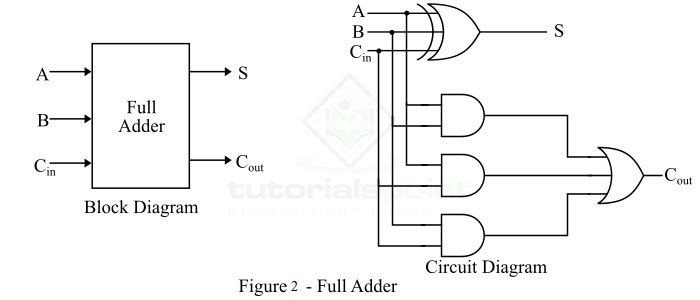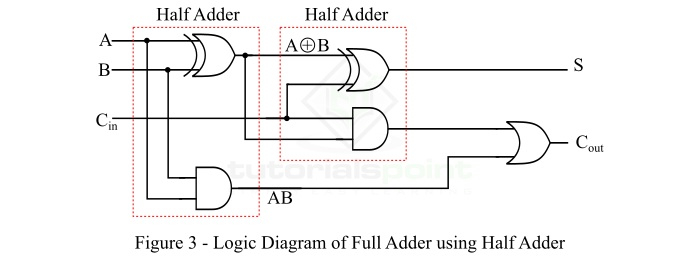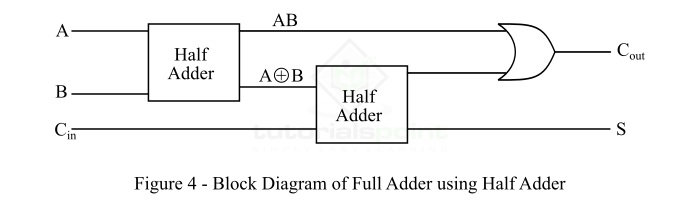In data processing, addition of operands is one of the most basic operations performed by different electronic devices like computers, calculators, etc. The electronic circuit that is designed to perform the addition of two or more numbers, more specifically binary numbers, is known as adder. As we know, the logic circuits use binary number system to perform the operations, hence the adder is also referred to as a binary adder.

## What is a Half Adder?

Half adder is a combinational logic circuit that is designed to add two binary digits. The half adder provides the output along with a carry (if any). The half adder circuit can be designed by connecting an XOR gate and one AND gate. It has two input terminals and two output terminals for sum (S) and carry (C). The block diagram and circuit diagram of a half adder are shown in Figure-1.In the half adder, the output of the XOR gate is the sum of two bits and the output of the AND gate is the carry bit. However, in the half-adder circuit, the carry obtained in one addition will not be forwarded in the next addition.

The output equation of the half adder are,

$$\mathrm{Sum,S=A\bigoplus B}$$

$$\mathrm{Carry,𝐶=A\cdot B}$$

## What is a Full Adder?

Full adder is also a combinational logic circuit that can add two binary digits (bits) and a carry bit, and produces a sum bit and a carry bit as output.

In other words, a combinational circuit which is designed to add three binary digits and produces two outputs (sum and carry) is known as a full adder. Thus, a full adder circuit adds three binary digits, where two are the inputs and one is the carry forwarded from the previous addition. The block diagram and circuit diagram of the full adder are shown in Figure-2.It is clear that the logic circuit of a full adder consists of one XOR gate, three AND gates and one OR gate, which are connected together as shown in Figure-2. Here, A and B are the input bits, $C_{in}$ is the carry from previous addition, S is the sum bit, and $C_{out}$ is the output carry bit.

The output equations of the full adder are,

$$\mathrm{Sum,S=A\bigoplus B\bigoplus C_{in}}$$

$$\mathrm{Carry,C_{out}=Ab+AC_{in}+BC_{in}}$$

Now, let us discuss the realization of the full adder using half adders

The logic diagram of the full adder using two half adders is shown in Figure-3:The block diagram of a full adder using two half adders is shown in Figure-4.From the logic diagram of the full adder using half adders, it is clear that we require two XOR gates, two AND gates and one OR gate for the implementation of a full adder circuit using half-adders.

However, the implementation of full adder using half adder has a major disadvantage that is the increased propagation delay. That means, the input bits must propagate through several gates in succession that increases the total propagation delay of the full adder circuit.